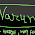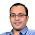## Nov 11, 2009

### Polya's Urn Problem

Puzzle: There are two urns with one ball each. Each of subsequent n-2 balls is placed into one of these urns, with probability proportional to the number of balls already in that urn. What is the expected number of balls in the smaller sized urn?

Source:P. Winkler's Puzzles book. (Chapter: Probability).

Solution:
Highlight the part between the * symbols for the answer.
*This problem can be reformulated as the following problem.

Suppose I have a stack of black cards and one red card. Initially I take red card in my hand. Now I add black cards randomly between any two cards (so, initially its either above or below red). Note that the probability that I add the card above the red card, when x-1 is the number of cards above red and y-1 is the number of cards below red is x/(x+y). Let the problem be if red card is dividing the black cards into two sets, what is the expected number of black cards in the smaller section. So, we see that the two problems are equivalent.

Now this way, we are getting all possible combinations in which one red and n black cards can be mixed, we see that the probability that the red card is at height h is independent of h. So, the probability that the smallest urn contains n/2 balls or 1 ball (or any number of balls between 1 and n/2) are all same. So, expected number of balls in the smaller urn is asymptotically n/4. :)

I am happy!! Happy Happy Joy Joy!! *

#### 26 comments:

1.just came upon your blog. interesting puzzles. could be more interesting if you waited a day or 2 between posting the problem and the solution. you might receive a lot of other interesting solutions and approaches that way.

2.Thanx Ram...
Will try that from the next set :)

3.here's one for you.

12 chinese women in a straight line one behind the other. someone comes and places a pin in the hair of each woman in the line. the pin can either be black or white, with equal possibility.the 12th woman in the line can see the pins of all the other 11 women. the 11th in the line can see the pins of the 10 women in front of her, and so on such that the first woman in the line can see noone's pin. starting from the 12th woman, each woman has to call out the color of her own pin (everyone else can hear). what strategy can they follow to maximize the number of correctly called out pin colors? what is the minimum number of correct pins guaranteed by such a strategy?

other than calling out 'black' or 'white' no other form of communication is allowed. no pauses, intonations, etc. can be used to convey information.

4.@Ram .. I posted this puzzle a few days back!!

11 women can identify the pin... Except the last one, everyone can.. Last one could tell the parity of white pins ahead of her... Others can then find their pin.. :)
Reference: http://pratikpoddarcse.blogspot.com/2009/08/cap-puzzle.html :)
Thanx.. Gimme more.. ;)

5.i didn't notice you already had it - my bad. its one of my favorites.

here's another:
10-11-12-13-14-20-22-101-?

6.Thanx Ram...

thats an easy one.. :)
next is 1010 and then is 1111111111 :)

1.Please tell me the explanation for this one next term in sequence 10-11-12-13-14-20-22-101-?

2.what is the explanation for next term in 10-11-12-13-14-20-22-101-?

3.Represent 10 in base 10,9,8,7,6,5,4,3,2,1

7.Good one! Here's another:

300 people waiting to enter a movie theatre in the order of their seat number (their seat numbers are from 1 to 300). first guy in line ignores seat number and just sits randomly on some seat. everyone else sits in their assigned number if available, and some random seat if not. what is the prob that the 300th guy gets to sit in his own seat?

8.The answer is 1/2 for any number of people :)

9.perfect answer, but tell me your solution (other than induction).

10.Induction of course does it..

The other solution is as follows:

Observe that when the 300th passenger finally boards, the seat remaining would be either the one assigned to him or the one assigned to the first passenger. Every other seat has either been taken by the rightful owner or by someone who reached there first. Since there is no pref what so ever to any seat, the prob is 1/2.
:)

11.very impressive. just a nitpick: it should be 'since there is no pref what so ever to EITHER OF THESE 2 SEATS'. other seats do have preferences from their respective assigned persons. only these two don't (so far).

here's another:
A. B & C live together and share everything equally. one day A brings home 5 logs of wood, B brings 3 logs and C brings none. then they use the wood to cook together and share the food. since C did not bring any wood, he gives \$8 instead. how much to A and how much to B?

12.Thanx for that correction..

Solution to the woods problem:
Since each person consumed 8/3 woods. A gave 5-8/3 = 7/3 woods to C and B gave 3-8/3 = 1/3 woods to C.
So, Out of the 8 dollars, A gets 7 and B gets 1 :)

13.very nice :)

last one for now...

there's a duck swimming in a circular lake with a wolf at the edge. wolf won't enter water. duck in water is slower than wolf on land. duck on land is faster than the wolf on land. given the lake diameter 'd' and wolf speed 'w', what is the minimum speed of the duck in water so that he can escape? what should be his escape strategy?

14.w/4 :)

This is a real difficult one. But (being honest), I solved this problem 3-4 years back.

The solution is interesting indeed.

Duck moves in a circle of radius 1/4. Here 2*pi*1/4.
Since here the duck would complete the circle as fast as the wolf, duck can get "diametrically" opposite to the wolf. And after that, duck has to cover distance 3/4 while wolf has to cover pi*1 which he will not be able to do. So, a speed just greater than w/4 would work. :)

Thanx for the interesting puzzles :)

15.To be exact, let the speed be w/k. Let the radius at which duck moves be r.

2pi*r*k > 2pi*1
So, rk > 1

Now, (1-r)*k < pi
Keeping rk = 1
k = pi+1
So, the minimum speed is w/(4.14)
:)

16.Impressed with your solution for 300 people theater problem. Specifically with the use of symmetry in discerning that probability should be 1/2.
Having an eye on symmetry reduces work that we need to do, Can you give me any pointers for developing intuition for symmetry. I know i am asking an open ended question, but any help will be appreciated.
Thanks,
Giridhar.

PS : Wordpress.com has LATEX Support. Many are converting from blogger.com especially for this reason. It will make life easier posting math related things.

17.@gridhar..
Thanx..
The question asked by you is really open ended.. :P

Many "good" probability questions are solved by symmetries. You might want to read about the major probability paradoxes. http://en.wikipedia.org/wiki/Category:Probability_theory_paradoxes

Of course, the argument given for the 300th problem was really involved and not trivial. I don't know if there are problems in which similar argument gives a solution.

I don't think I have helped you. Sorry.

You can post some pointers if you find. You can also post interesting problems.

For the wordpress thing, the work is on. Will be up in a few days. Thanx.

18.Thanks Pratik.

Here is one question :
You have coins C_1, C_2, . . . , C_n . For each k, coin C_k is biased so that, when tossed, it has probability 1/(2k+1) of falling heads. If the n coins are tossed, what is the probability that number of heads is odd? Express the answer as a rational function of n ? ( Boring Question ?? )

It is easy to guess and prove using Mathematical Induction.
You can find one non-Inductive solution
@ http://connect2ppl.wordpress.com/

19.No math question can be boring :P

Not so difficult question. I could come up with the answer n/(2n+1) using induction.

But awesome solution using probability-generating function. Never knew that something like that exists. Thanks a lot.

Solution to the problem at http://connect2ppl.wordpress.com/2009/12/08/calculating-probability-via-generating-functions/

20.In response to your comment on http://gurmeetsingh.wordpress.com/2008/09/12/puzzle-polyas-urn-problem/

It is difficult to refute ur argument, hence let us take help of math ( in accordance with diophantine who said " equations are more intelligent than their creators " ) --
Let us represent the outcome of n-2 throws with sequence of length n-2 containing 1& 2's ( corresponding to the bin they land into ).
Let P(x, n-x) stand for the probability of having x balls in the first bin and n-x balls in the second bin.
Note that the probability of individual sample points containing x 1's and (n-x) 2's is each equal to [(x-1)!(n-x-1)!]/(n-1)!.
The number of sample points with x 1's and (n-x) 2's is equal to (n-2)!/[(x-1)!(n-x-1)!]
Multiplying these two results we get
P(x, n-x) = 1/(n-1).

In retrospect we see sequences with high probability are less in number and sequences with less probability are high in number, leveling out the things finally.

Thanks,
Giridhar.

21.Just so that people understand things:

We are talking about the Poyla's Urn problem only http://pratikpoddarcse.blogspot.com/2009/11/polyas-urn-problem.html

I wrote a comment on gurmeet's blog http://gurmeetsingh.wordpress.com/2008/09/12/puzzle-polyas-urn-problem/

@Giridhar.. Thanx for that.. great argument..

22.http://connect2ppl.wordpress.com/2009/12/08/calculating-probability-via-generating-functions/

Is it not an overkill?

I mean if you let K_{n} = (2n+1)*P{n}
And then it becomes

K_{n} = K_{n-1} + 1

Btw, generating functions are still the only way I know of for solving difference equations with more than one parameters. Is there any better way for solving something like
P(a,b) = k_{1} * P(a-1,b) + k_{2} * P(a-1, b-3)
where k_{1}, k_{2} are constants.

23.For Polya's Urn puzzle, can't we do it the following way :

Let E(n-1) be the expected number of balls in smaller urn.

E(n) = E(n-1)/n-1 * (1 + E(n-1)) + (1 - E(n-1)/n-1) * E(n-1);

On solving -> E(n-1) = n/n-1 * E(n-1)
Since E(3) = 1, therefore E(n) = n/3.

### Fraction Brainteaser

Source: Sent to me by Gaurav Sinha Problem: Siddhant writes a Maths test and correctly answers 5 out of 6 Arithmetic questions and 20...